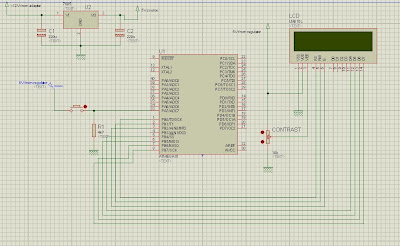## Saturday, March 31, 2012

### Simple Fun Morse Code decoder with AVR microcontroller

Hi people...let's have some fun with AVR micros.This is my first post on any hardware project based on microcontroller except programmer or programming hardware.It's just a fun project but can also be useful to those who practice morse code...Well by this time u know what that thing is...it's a simple morse code decoder with one button,ATmega16(not overkill,kind of superkill for anything like this,but at that time,that was easy to work with for me as I had a development board for 40pin avrs),LCD for showing output.

Unfortunately,that circuit is a history now so I cant post any image of it(Sorry folks).But I will post the schematic and a video I uploaded then to youtube(Though the video quality is...better see for yourselves).
Okay let's come to the point.Many of you know what morse code is...it's an old system of electrical communication where messages are sent as a combination of Dash(long duration beeps or current flow) and dots(shot beeps or current flow).This was used in morse telegraph communications systems.The morse code chart is given below for interested people from wikipedia:Though I didnt follow the timing issues then,only used the codes.

Now,why this old stuff using new things right???Well,answer is simple...mainly for fun and some skill testing also :) .
Here goes my schematic:Circuit is pretty simple...just one button for taking input.

I had to add some extra things for displaying to LCD and clearing the LCD etc. such as a 7sec delay after entering the code to show the result on LCD.

The source code was written in Bascom AVR.Its a pretty cool compiler and easy to work with and awesome for string handling and code optimization.The source code is not BIG so It is posted here.

THE CODE:

'8th pin of PORTA used for input

\$regfile = "m16def.dat"

\$crystal = 8000000

\$lib "lcd4busy.lib"
'defining LCD pins
Const _lcdport = Portb
Const _lcdddr = Ddrb
Const _lcdin = Pinb
Const _lcd_e = 2
Const _lcd_rw = 1
Const _lcd_rs = 0
Dim A As Integer 'the delay counter
Dim Bttn_pressed As Integer 'variable to check if the button has been pressed before when released
Dim Mrsc As String * 32 'string for holding the morse code input
Dim Strf As String * 32 'string for holding the processed output
Dim X As Integer 'LCD upper line character position

Mrsc = ""
Strf = ""
Ddra = 0
Cursor Off
Cls
X = 1
Do
If Pina.7 = 1 Then
A = A + 1
Bttn_pressed = 1
Waitms 500
Elseif Pina.7 = 0 Then
If Bttn_pressed = 1 Then
If A = 1 Then
A = 0
Bttn_pressed = 0
End If
If A > 1 And A < 3 Then
Upperline
Locate 1 , X
Lcd "."
Mrsc = Mrsc + "."
A = 0
'Waitms 10
Bttn_pressed = 0
X = X + 1
Elseif A >= 3 And A < 6 Then
Upperline
Locate 1 , X
Lcd "_"
Mrsc = Mrsc + "_"
A = 0
Bttn_pressed = 0
X = X + 1
'Waitms 10

Elseif A >= 6 And A < 12 Then
Select Case Mrsc
Case "._"
Strf = Strf + "A"
Case "_..."
Strf = Strf + "B"
Case "_._."
Strf = Strf + "C"
Case "_.."
Strf = Strf + "D"
Case "."
Strf = Strf + "E"
Case ".._."
Strf = Strf + "F"
Case "__."
Strf = Strf + "G"
Case "...."
Strf = Strf + "H"
Case ".."
Strf = Strf + "I"
Case ".___"
Strf = Strf + "J"
Case "_._"
Strf = Strf + "K"
Case "._.."
Strf = Strf + "L"
Case "__"
Strf = Strf + "M"
Case "_."
Strf = Strf + "N"
Case "___"
Strf = Strf + "O"
Case ".__."
Strf = Strf + "P"
Case "__._"
Strf = Strf + "Q"
Case "._."
Strf = Strf + "R"
Case "..."
Strf = Strf + "S"
Case "_"
Strf = Strf + "T"
Case ".._"
Strf = Strf + "U"
Case "..._"
Strf = Strf + "V"
Case ".__"
Strf = Strf + "W"
Case "_.._"
Strf = Strf + "X"
Case "_.__"
Strf = Strf + "Y"
Case "__.."
Strf = Strf + "Z"
Case "____"
Strf = Strf + " "
End Select
Cls
Lowerline
Lcd Strf
Mrsc = ""
A = 0
X = 1
Bttn_pressed = 0

Elseif A >= 15 Then
Cls
Bttn_pressed = 0
Mrsc = ""
Strf = ""
A = 0
X = 1
End If
End If
End If
Loop
End

Code has been commented so It should be easy to understand...

Here is the (Sorry again for quality)...well...video...:)

If you need some information...tell me.Have fun!!!